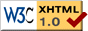# Draft Proposal for UCS-E Specification

Introduction | Properties | Details | Examples | Discussion | UCS-X Home Page

Authors: Tom Bishop (tbishop@wenlin.com) and Richard Cook (rscook@wenlin.com).

Updated October, 2009.

Please Note: This is not a finalized specification. It is still at the "draft proposal" stage and may change.

## 1. Introduction

The name of this specification is "UCS-E".

• UCS = Universal Character Set (or Superset).
• E = exa- = 1018 in the International System of Units.

UCS-E enables extension of the Universal Character Set to support more than 9x1018 (nine times ten to the eighteenth power) characters, with code points up to U+7FFFFFFFFFFFFFFF. It is a member of the UCS-X family of specifications. It provides three encoding forms, UTF-E-8, UTF-E-16, and UTF-E-32, which are compatible extensions of UTF-8, UTF-16, and UTF-32, respectively.

## 2. Properties

UCS-E preserves and extends all the useful properties of UCS-G.

## 3. Details

The set of UCS-E code points is the range U+0000..U+7FFFFFFFFFFFFFFF (fifteen F's). The number of code points is 263. As in Unicode, U+D800..U+DFFF (211 = 2,048 "surrogate code points") are excluded from the set of USV (UCS scalar values). Hence, the number of UCS-E scalar values is (exactly) 263 - 211 = 9,223,372,036,854,773,760 = (approximately) 9x1018 (nine quintillion).

UCS-E specifies three encoding forms (using 8-bit, 16-bit, and 32-bit units) that each associate a unique code with each USV. Detailed specifications are provided for the three encoding forms. Please see:

## 4. Examples

```USV = U+0041 (the letter 'A')
UTF-E-8  = 41
UTF-E-16 = 0041
UTF-E-32 = 00000041

USV = U+5B57 (the Chinese/Japanese/Korean character '字')
UTF-E-16 = 5B57
UTF-E-32 = 00005B57

USV = U+10FFFF (the last USV in the Unicode Standard)
UTF-E-8  = F4 8F BF BF
UTF-E-16 = DBFF DFFF
UTF-E-32 = 0010FFFF

USV = U+110000 (the first USV beyond the U+10FFFF limit)
UTF-E-8  = F4 90 80 80
UTF-E-16 = DC04 DE80 DE00
UTF-E-32 = 00110000

USV = U+7FFFFFFF (the last USV in the original ISO 10646 standard)
UTF-E-8  = FD BF BF BF BF BF
UTF-E-16 = DD0F DFFF DFFF DFFF
UTF-E-32 = 7FFFFFFF

USV = U+80000000 (the first USV beyond the original ISO 10646)
UTF-E-8  = FE 82 80 80 80 80 80
UTF-E-16 = DD10 DE00 DE00 DE00
UTF-E-32 = 80000000

USV = U+123456789
UTF-E-8  = FE 84 A3 91 96 9E 89
UTF-E-16 = DD24 DED1 DEB3 DF89
UTF-E-32 = F0000012 E3456789

USV = U+7FFFFFFFFFFFFFFF (the last USV supported by UCS-E)
UTF-E-8 = FF 80 87 BF BF BF BF BF BF BF BF BF BF
UTF-E-16 = DDF0 DFFF DFFF DFFF DFFF DFFF DFFF DFFF
UTF-E-32 = FF00007F EFFFFFFF EFFFFFFF
```

More examples are shown in the individual specifications for UTF-E-8, UTF-E-16, and UTF-E-32.

## 5. Discussion

The following graph compares the code lengths of UTF-E-8, UTF-E-16, and UTF-E-32. Note that each of the three forms is most efficient for certain ranges, and overall efficiency is similar.For implementation, applications, references, etc., please see the UCS-X page.